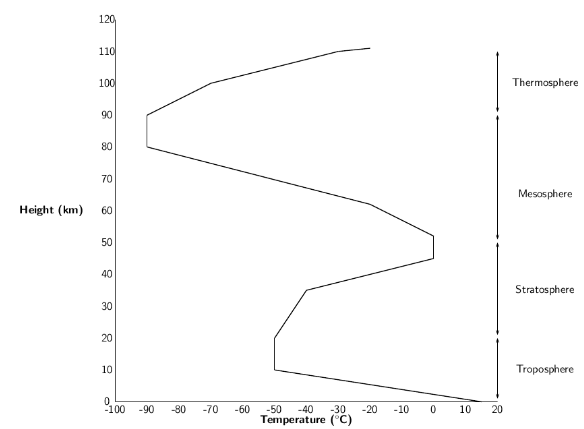# Composition and structure  (Page 2/3)

 Page 2 / 3
Earth's atmosphere

The Earth's atmosphere is a layer of gases that surround the planet, and which are held there by the Earth's gravity. The atmosphere contains roughly 78.1% nitrogen, 20.9% oxygen, 0.9% argon, 0.038% carbon dioxide, trace amounts of other gases, and a variable amount of water vapour. This mixture of gases is commonly known as air. The atmosphere protects life on Earth by absorbing ultraviolet solar radiation and reducing temperature extremes between day and night.

## The structure of the atmosphere

The earth's atmosphere is divided into different layers, each with its own particular characteristics ( [link] ).A generalised diagram showing the structure of the atmosphere and the changing temperatures up to a height of 110 km

## The troposphere

The troposphere is the lowest level in the atmosphere, and it is the part in which we live. The troposphere varies in thickness, and extends from the ground to a height of about 7km at the poles and about 18km at the equator. An important characteristic of the troposphere is that its temperature decreases with an increase in altitude. In other words, as you climb higher, it will get colder. You will have noticed this if you have climbed a mountain, or if you have moved from a city at a high altitude to one which is lower; the average temperature is often lower where the altitude is higher. This is because the troposphere is heated from the 'bottom up'. In other words, places that are closer to the Earth's surface will be warmer than those at higher altitudes. The heating of the atmosphere will be discussed in more detail later in this chapter.

The word troposphere comes from the Greek tropos , meaning turning or mixing . The troposphere is the most turbulent (or agitated) part of the atmosphere and is the part where our weather takes place. Weather is the state of the air at a particular place and time e.g. if it is warm or cold, wet or dry, and how cloudy or windy it is. Generally, jet aircraft fly just above the troposphere to avoid all this turbulence.

## The stratosphere

Above the troposphere is another layer called the stratosphere , where most long distance aircraft fly. The stratosphere extends from altitudes of 10 to 50km. If you have ever been in an aeroplane and have looked out the window once you are well into the flight, you will have noticed that you are actually flying above the level of the clouds. As we have already mentioned, clouds and weather occur in the troposphere, whereas the stratosphere has very stable atmospheric conditions and very little turbulence. It is easy to understand why aircraft choose to fly here!

The stratosphere is different from the troposphere because its temperature increases as altitude increases. This is because the stratosphere absorbs solar radiation directly, meaning that the upper layers closer to the sun will be warmer. The upper layers of the stratosphere are also warmer because of the presence of the ozone layer . Ozone (O ${}_{3}$ ) is formed when solar radiation splits an oxygen molecule (O ${}_{2}$ ) into two atoms of oxygen. Each individual atom is then able to combine with an oxygen molecule to form ozone. The two reactions are shown below:

#### Questions & Answers

Why do vectors have arrows?
vector quantity has both direction and magnitude so arrows illustrate the direction of the vector
Mhleli
how to calculate net electric field at a point
what are controlled variables
Independent variables in an experiment which influence the outcome results
Boss
Such as temperature when dealing with ohms law
Boss
variables whose amount is determined by the person doing the experiment
Clemencia
how to calculate exodation number
Calculate relative atomic mass
what is chemical bonding?
Chemical Bonding is a mutual attraction between two atoms resulting from the simultaneous attraction between their nuclei and the outer elections
Sydney
How would you go about finding the resistance of an unknown resistor using only power supply, a voltmeter and a known resistance
Lebona
who is the scientist who discovered electromagnetism
what happens to the galvanometer when the switch is closed ?
Zivele
how do we identify an oxidising or reducing agent in a reaction?
Zivele
hey
Banele
what is electricity
Formula for concentration
c=m/MV
Zipho
if given number of moles and volume , can use c=n/V
sibahle
Chemistry term three topic is stressing me out, I really need a huge help
Ziyanda
on what
Olebogeng
during a snooker competition ,a 200g ball A m moving with velocity va collide head on with a identical ball B that was at rest.A after the collision ball A remains at rest wile ball B moves on with a velocity of 4m/s? With what speed was ball a moving before the collision
a vector can be resolved into a horizontal component only
how to calculate normal force
how to calculate wavelength
Lizoh
Hello. How does a real gas behave under low temperature and high pressure?
does a vector quantity include force and distance?
yes
Itumeleng
what's the difference between a vector and a scalar?
Keaobaka
vector is the physical quantity with magnitude and direction Scalar is the Physical quantity with magnitude only
Bobo
Newton's second law of motion
Newton second law motin
Shife
Newton's second law of motion: When a resultant/net force acts on an object, the object will accelerate in the direction of the force at an acceleration directly proportional to the force and inversely proportional to the mass of the object.
Bobo
newtons third law of motion
Ayakha
when object A exert a force on object B object B SIMULTANEOUS exert a force of equal magnitude on object A
TshepisoBy Anindyo MukhopadhyayBy Abishek DevarajByBy